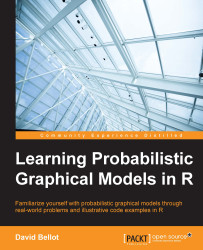•#### Learning Probabilistic Graphical Models in R#### Overview of this book

Probabilistic graphical models (PGM, also known as graphical models) are a marriage between probability theory and graph theory. Generally, PGMs use a graph-based representation. Two branches of graphical representations of distributions are commonly used, namely Bayesian networks and Markov networks. R has many packages to implement graphical models. We’ll start by showing you how to transform a classical statistical model into a modern PGM and then look at how to do exact inference in graphical models. Proceeding, we’ll introduce you to many modern R packages that will help you to perform inference on the models. We will then run a Bayesian linear regression and you’ll see the advantage of going probabilistic when you want to do prediction. Next, you’ll master using R packages and implementing its techniques. Finally, you’ll be presented with machine learning applications that have a direct impact in many fields. Here, we’ll cover clustering and the discovery of hidden information in big data, as well as two important methods, PCA and ICA, to reduce the size of big problems.
Learning Probabilistic Graphical Models in RCreditswww.PacktPub.comPrefaceFree Chapter
Probabilistic ReasoningExact InferenceLearning ParametersBayesian Modeling – Basic ModelsApproximate InferenceBayesian Modeling – Linear ModelsProbabilistic Mixture ModelsAppendixIndex## Introduction

In this chapter, we will learn how to make the computer learn about the parameters of a model. Our examples will use various datasets we will build ourselves or other datasets we will download from various websites. There are many datasets available online and we will use data from the UCI machine learning repository. These are made available by the Centre for Machine Learning and Intelligent Systems of the University of California, Irvine (UCI).For example, one of the most famous datasets is the Iris dataset where each data point in the dataset represents the characteristics of an iris plant. Different attributes are used such as the sepal length/width and petal length/width.

It is possible to download this dataset and store it into a `data.frame` in R as we will do most of the time. Each variable is in a column and we will use i.i.d data (or assume...